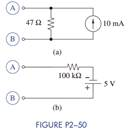Chat Now

# Using no more than four 1-kΩ resistors, show how the following equivalent resistors can be...

### Using no more than four 1-kΩ resistors, show how the following equivalent resistors can be...

1. Using no more than four 1-kΩ resistors, show how the following equivalent resistors can be constructed: 2 kΩ, 500 Ω, 1:5 kΩ, 333 Ω, 200 Ω, and 400 Ω.

2. Do a source transformation at terminals A and B for each practical source in Figure P2−50.manish jayant 28-Jul-2020Get solution